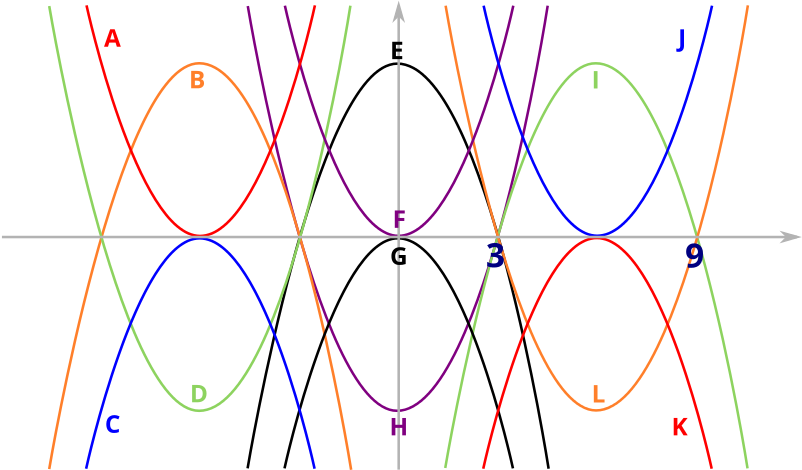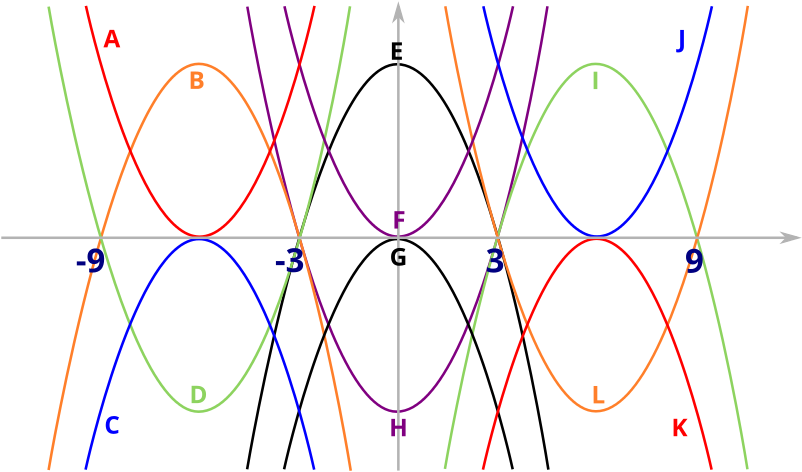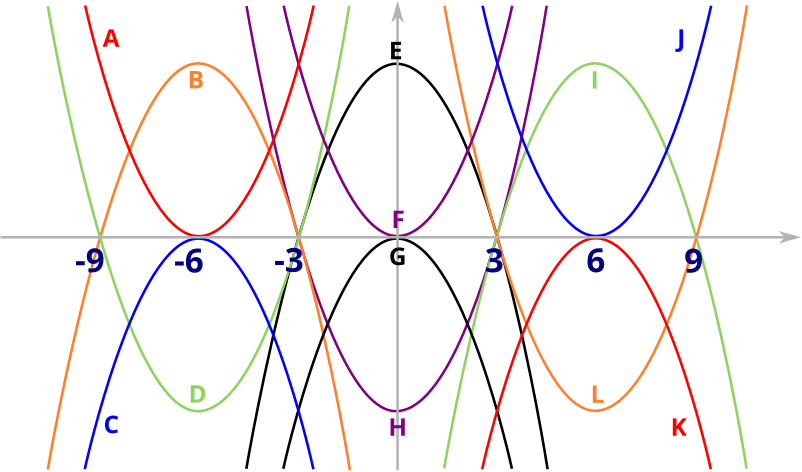Package of problems

## Solution

Given that two of the parabolas have the equations $y=x^2-12x+27$ and $y=-x^2+12x-36,$ can you find the equations of the other parabolas?

Here is a summary of the equations that you should have found.

Parabola Equation
A $y=x^2+12x+36$
B $y=-x^2-12x-27$
C $y=-x^2-12x-36$
D $y=x^2+12x+27$
E $y=-x^2+9$
F $y=x^2$
G $y=-x^2$
H $y=x^2-9$
I $y=-x^2+12x-27$
J $y=x^2-12x+36$
K $y=-x^2+12x-36$
L $y=x^2-12x+27$

Here is a way to find the equations of the parabolas.

We will first look at the equation

$\begin{equation} y=x^2-12x+27=(x-3)(x-9).\label{eq:1} \end{equation}$

The graph of this equation will intersect the $x$-axis at $x=3$ and $x=9$. The are only two parabolas in the picture that intersect the positive $x$-axis twice: parabolas I and L, which are symmetrical about the $x$-axis, which corresponds to the map $y\mapsto -y$. Since the coefficient of $x^2$ is positive, we know the parabola must look like $\cup$ rather than $\cap$, so the equation $\eqref{eq:1}$ must correspond to parabola L. But we now also know what the equation of parabola I is: $y=-x^2+12x-27$.We can now mark some points on the $x$-axis

We can also see from the picture that parabola B corresponds to parabola I reflected in they $y$-axis. This reflection corresponds to the map $x\mapsto -x$, so we can see that the equation of parabola B is $y=-(-x)^2+12(-x)-27=-x^2-12x-27$. Similarly, parabola D is parabola L reflected in the $y$-axis, so parabola D has the equation $y=x^2+12x+27$.We can now mark more points on the $x$-axis

Now let’s think about the equation

$\begin{equation} y=-x^2-12x-36=-(x+6)^2.\label{eq:2} \end{equation}$

The parabola corresponding to $\eqref{eq:2}$ has a double root at $x=-6$, so the top of the parabola must just touch the $x$-axis (the coefficient of $x^2$ is $-1$ so it looks like $\cap$ rather than $\cup$). We know where $(-3,0)$ and $(-9,0)$ are on the picture because we know that parabola B and D intersect the $x$-axis at these points. Parabola A and C both have a double root at $x=-6$, so from their shapes we see that equation $\eqref{eq:2}$ must be the equation of parabola C, and that the equation of parabola A, its reflection in the $x$-axis, must have the equation $y=(x+6)^2=x^2+12x+36$.

We can see from the picture that parabolas J and K are parabolas A and C reflected in the $y$-axis respectively. So parabola J must have the equation corresponding to parabola A under the transformation $x\mapsto -x$, which is $y=x^2-12x+36$. Similarly, parabola K has the equation $y=-x^2+12x-36$.We can now mark all of the important points on the $x$-axis

We still need to find the equations of parabolas E, F, G and H. From the picture, we see that E and H both intersect the $x$-axis at the points $(3,0)$ and $(-3,0)$, so they look like $y=\pm\alpha(x+3)(x-3)$, for some $\alpha>0$. From their shapes, we see that parabola E has equation $y=-\alpha(x+3)(x-3)=-\alpha x^2+9\alpha$ and parabola H has equation $y=\alpha x^2-9\alpha$. To find the value of $\alpha$, we compare to parabola I, which has the same height. The peak of parabola I is in the middle of its two roots, since a parabola is symmetrical. So the height of parabola I, and therefore of E, is $9$. Since the peak of parabola E is at $x=0$, we require that $9=0^2\alpha+9\alpha$, and so $\alpha=1$.

Finally, parabolas F and G both have a double root at $x=0$, which means they have the form $y=\pm \alpha x^2$, for some $\alpha>0$. Again looking at their shapes, we see that parabola F has equation $y=\alpha x^2$ and parabola G has equation $y=-\alpha x^2$. To find the value of $\alpha$, notice that parabola F is a translation of parabola J along the $x$-axis by $6$ in the negative direction. So the equation of parabola F must be that of parabola J evaluated at $(x+6)$ instead of $x$, and so is $y=(x+6)^2-12(x+6)+36=x^2+12x+36-12x-72+36=x^2$. So $\alpha=1$.